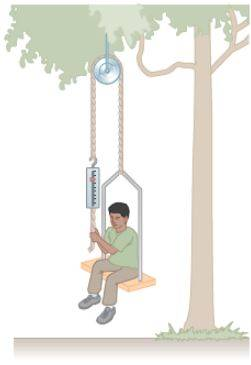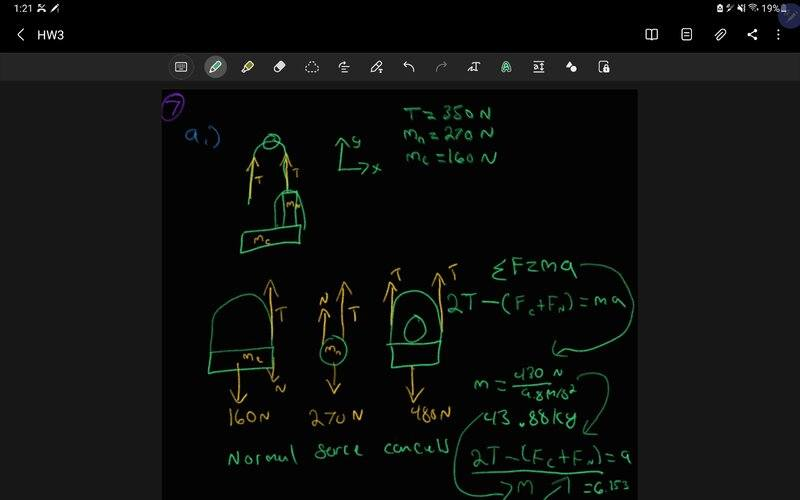# HW help: Tension/force/acceleration question

• brandonp620
What forces act on Nick now?The tension in the rope is the same, but the force exerted by the rope on the Nick + chair system is not.f

#### brandonp620

Summary:: An inventive child named Nick wants to reach an apple in a tree without climbing the tree. Sitting in a chair connected to a rope that passes over a frictionless pulley (see figure below), Nick pulls on the loose end of the rope with such a force that the spring scale reads 350 N. Nick's true weight is 270 N, and the chair weighs 160 N. Nick's feet are not touching the ground. Use g=9.8 m/s^2.
a = 6.153 m/s^2
magnitude nick on chair 89.52N
Friend pulling pully: ? m/s^2

An inventive child named Nick wants to reach an apple in a tree without climbing the tree. Sitting in a chair connected to a rope that passes over a frictionless pulley (see figure below), Nick pulls on the loose end of the rope with such a force that the spring scale reads 350 N. Nick's true weight is 270 N, and the chair weighs 160 N. Nick's feet are not touching the ground. Use g=9.8 m/s^2.

Find Nick's acceleration, using upward as positive: a = 6.153 m/s^2

Find the magnitude of the force Nick exerts on the chair: 89.52N

Instead Nick hands the rope with the scale to his friend Barney, who stands on the ground. Barney pulls on the rope so that the spring scale again reads 350 N. What is Nick's acceleration now, again using upward as positive.: ? m/s^2Not sure what changes in the last question T would still equal the same. Not sure what forces changes in the free body diagram, would deeply appreciate any help with the problem.

Not sure what changes in the last question T would still equal the same. Not sure what forces changes in the free body diagram, would deeply appreciate any help with the problem.
The tension in the rope is the same, but the force exerted by the rope on the Nick + chair system is not. Do you see why?

•brandonp620
Not sure what changes in the last question T would still equal the same. Not sure what forces changes in the free body diagram, would deeply appreciate any help with the problem.

The tension in the rope is the same, but the force exerted by the rope on the Nick + chair system is not. Do you see why?
I do not, It would still have the Normal force, and mg and Tension would still be in the same direction. So I'm a little lost. Would there only be one tension force?

•